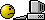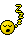## Five minutes gone - power.tsd.2m() arose [Two-Stage / GS Designs]

Dear Helmut, dear All,

❝ ❝ The adjusted α showed (well, in simulations…) that the TIE is controlled if we follow the frameworks exactly, i.e., initiate the second stage only if necessary based on the interim and with the re-estimated sample size. If we increase the sample size, the chance of passing BE increases and hence, the TIE.

❝ Wait, wait ... I will be back in 5 minwith some quick shoot code to verify your claim.

5 minutes are gone.
See Power2Stage on Github. Function power.tsd.2m().
It's pure Potvin B, abbreviated with implicit power monitoring, i.e. via sample size estimation, which gives n2=0 in case of 'enough' power.
What's missing at the moment is some sort of correlation between the PK metrics analogous to what is possible in power.2TOST() with the argument rho. Here I don't know at the moment at which place it comes into play. Suggestions are welcome.
The function is not public at the moment. Not in the mood to document.

Example:
# install from GitHub via
# devtools::install_github("Detlew/Power2Stage")

library(Power2Stage) # Cmax not BE, but AUC assumed BE Power2Stage:::power.tsd.2m(CV=c(0.3, 0.2), theta0=c(1.25, 0.95), n1=28)$pBE  0.043414 # Cmax assumed BE, but AUC not BE Power2Stage:::power.tsd.2m(CV=c(0.3, 0.2), theta0=c(0.95, 1.25), n1=28)$pBE  0.031875 # Cmax not BE, AUC also not BE Power2Stage:::power.tsd.2m(CV=c(0.3, 0.2), theta0=c(1.25, 1.25), n1=28)\$pBE  0.001452

Take the results with a grain of salt. Don't know how to validate. Suggestions here also welcome.
But I think the results look plausible.

Edited numbers after removing a little bug.

Regards,

DetlewIng. Helmut Schütz